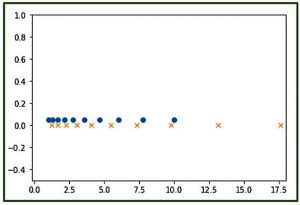Related Articles

# numpy.logspace() in Python

• Last Updated : 09 Jul, 2021

The numpy.logspace() function returns number spaces evenly w.r.t interval on a log scale.
Syntax :

```numpy.logspace(start,
stop,
num = 50,
endpoint = True,
base = 10.0,
dtype = None)```

Parameters :

Attention geek! Strengthen your foundations with the Python Programming Foundation Course and learn the basics.

To begin with, your interview preparations Enhance your Data Structures concepts with the Python DS Course. And to begin with your Machine Learning Journey, join the Machine Learning - Basic Level Course

```-> start    : [float] start(base ** start) of interval range.
-> stop     : [float] end(base ** stop) of interval range
-> endpoint : [boolean, optional]If True, stop is the last sample. By default, True
-> num      : [int, optional] No. of samples to generate
-> base     : [float, optional] Base of log scale. By default, equals 10.0
-> dtype    : type of output array```

Return :

`-> ndarray`

Code 1 : Explaining the use of logspace()

## Python

 `# Python Programming illustrating``# numpy.logspace method` `import` `numpy as geek` `# base = 11``print``(``"B\n"``, geek.logspace(``2.0``, ``3.0``, num``=``5``, base ``=` `11``))` `# base = 10``print``(``"B\n"``, geek.logspace(``2.0``, ``3.0``, num``=``5``))` `# base = 10, dtype = int``print``(``"B\n"``, geek.logspace(``2.0``, ``3.0``, num``=``5``, dtype ``=` `int``))`

Output :

```B
[  121.           220.36039471   401.31159963   730.8527479   1331.        ]
B
[  100.           177.827941     316.22776602   562.34132519  1000.        ]
B
[ 100  177  316  562 1000]```

Code 2 : Graphical Representation of numpy.logspace() using matplotlib module – pylab

## Python

 `# Graphical Representation of numpy.logspace()``import` `numpy as geek``import` `pylab as p` `# Start = 0``# End = 2``# Samples to generate = 10``x1 ``=` `geek.logspace(``0``, ``1``, ``10``)``y1 ``=` `geek.zeros(``10``)` `# Start = 0.1``# End = 1.5``# Samples to generate = 12``x2 ``=` `geek.logspace(``0.1``, ``1.5``, ``12``)``y2 ``=` `geek.zeros(``12``)` `p.plot(x1, y1``+``0.05``, ``'o'``)``p.xlim(``-``0.2``, ``18``)``p.ylim(``-``0.5``, ``1``)``p.plot(x2, y2, ``'x'``)`

Output :Note :
These NumPy-Python programs won’t run on onlineID, so run them on your systems to explore them
Similiar methods :

My Personal Notes arrow_drop_up